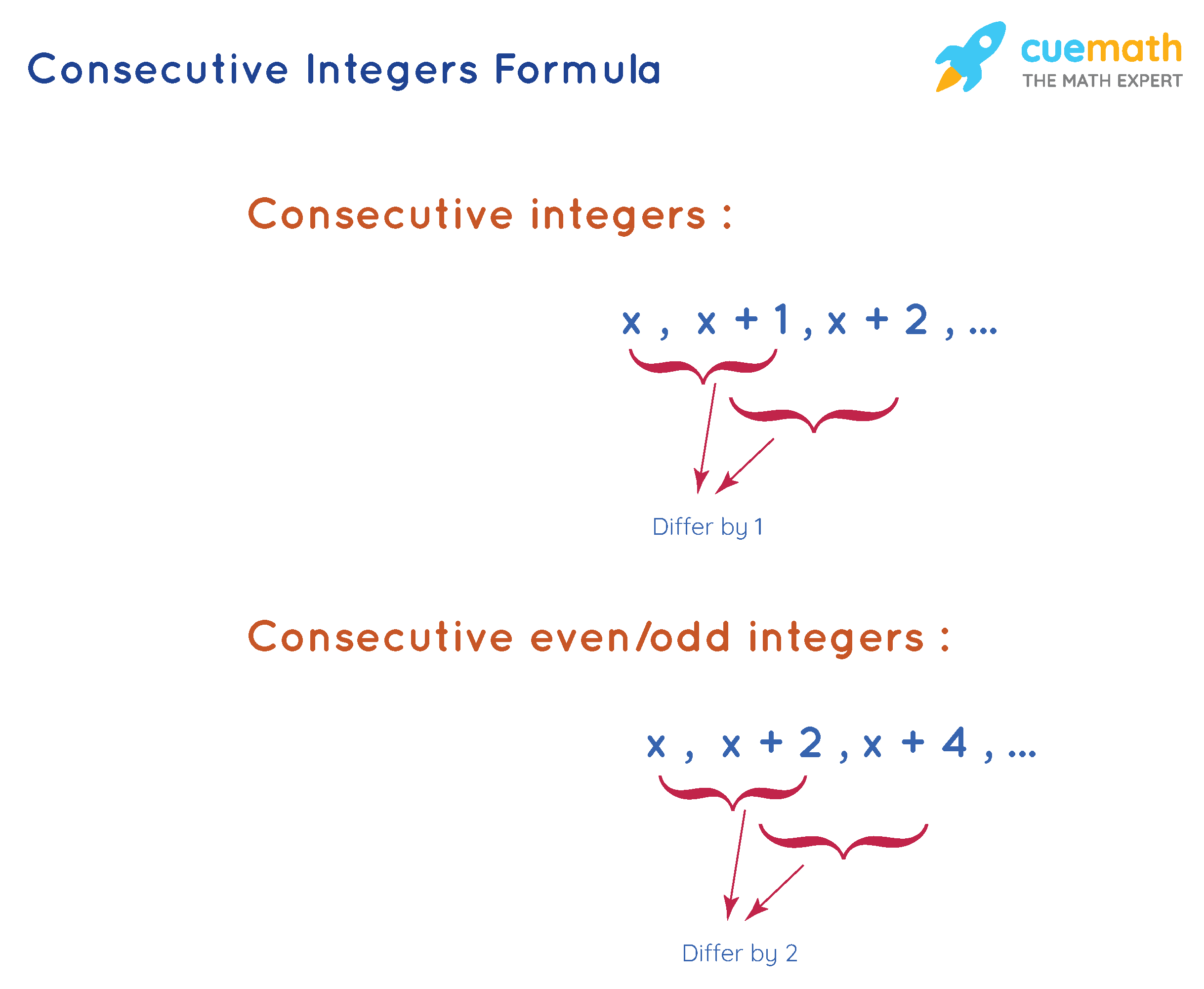# Consecutive Integers Formula

The consecutive integers are integers that follow each other in increasing order. Consecutive integers formula helps in finding consecutive integers for any given number, or to check whether a set of integers are consecutive or not.

Examples of Consecutive Integers

• 0, 1, 2, 3, 4, ...
• -3, -2, -1, 0, 1, ....
• -12, -11, -10, ...

We can see that each integer in any list of consecutive integers is obtained by adding 1 to its previous integer. Thus, two consecutive integers differ by 1.

Let us understand the consecutive integers formula using solved examples in the following sections.

## What is Consecutive Integers Formula?

Using the above explanation, we conclude that the consecutive integers are of the form:

x, x + 1, x + 2, x + 3, ....

where,

x is an integer, and

x + 1, x + 2, .. are successive consecutive integers in sequence.

In a problem involving consecutive integers, we assume the first integer to be x and the subsequent integers can be obtained by adding 1 to the previous integer.

We know that two consecutive even numbers (or) two consecutive odd numbers differ by 2. So any two consecutive even numbers (or) consecutive odd numbers are of the form:

x, x + 2, x + 4, ...

where,

x is an integer, and

x + 2, x + 4, .. are successive even/odd consecutive integers in sequence.## Solved Examples Using Consecutive Integers Formula

### Find the set of three consecutive integers whose sum is 78.

Solution:

To find: Set of three consecutive integers whose sum is 78.

Using the formula of consecutive integers, we can assume the three consecutive integers to be x, x + 1, and x + 2.

Their sum is given to be 78. So we get the equation:

x + (x + 1) + (x + 2) = 78

3x + 3 = 78

Subtracting 3 from both sides,

3x = 75

Dividing both sides by 3,

x = 25

So the three consecutive integers are:

x = 25

x + 1 = 25 + 1 = 26

x + 2 = 25 + 2 = 27

Answer: The required integers are 25, 26, and 27.

### Find three consecutive odd integers following the number -11.

Solution:

To find: Three consecutive odd integers following number -11.

We know that the consecutive odd integers differ by 2 and are of form x, x + 2, x + 4, ...

Let us assume that x = -11.

Then three consecutive odd integers of x are

x + 2 = -11 + 2 = -9

x + 4 = -11 + 4 = -7

x + 6 = -11 + 6 = -5

Answer: The three consecutive integers of -11 are -9, -7, and -5.